The point geom is used to create scatterplots. The scatterplot is most useful for displaying the relationship between two continuous variables. It can be used to compare one continuous and one categorical variable, or two categorical variables, but a variation like geom_jitter(), geom_count(), or geom_bin2d() is usually more appropriate. A bubblechart is a scatterplot with a third variable mapped to the size of points.

## Usage

geom_point(
mapping = NULL,
data = NULL,
stat = "identity",
position = "identity",
...,
na.rm = FALSE,
show.legend = NA,
inherit.aes = TRUE
)

## Arguments

mapping

Set of aesthetic mappings created by aes(). If specified and inherit.aes = TRUE (the default), it is combined with the default mapping at the top level of the plot. You must supply mapping if there is no plot mapping.

data

The data to be displayed in this layer. There are three options:

If NULL, the default, the data is inherited from the plot data as specified in the call to ggplot().

A data.frame, or other object, will override the plot data. All objects will be fortified to produce a data frame. See fortify() for which variables will be created.

A function will be called with a single argument, the plot data. The return value must be a data.frame, and will be used as the layer data. A function can be created from a formula (e.g. ~ head(.x, 10)).

stat

The statistical transformation to use on the data for this layer, either as a ggproto Geom subclass or as a string naming the stat stripped of the stat_ prefix (e.g. "count" rather than "stat_count")

position

Position adjustment, either as a string naming the adjustment (e.g. "jitter" to use position_jitter), or the result of a call to a position adjustment function. Use the latter if you need to change the settings of the adjustment.

...

Other arguments passed on to layer(). These are often aesthetics, used to set an aesthetic to a fixed value, like colour = "red" or size = 3. They may also be parameters to the paired geom/stat.

na.rm

If FALSE, the default, missing values are removed with a warning. If TRUE, missing values are silently removed.

show.legend

logical. Should this layer be included in the legends? NA, the default, includes if any aesthetics are mapped. FALSE never includes, and TRUE always includes. It can also be a named logical vector to finely select the aesthetics to display.

inherit.aes

If FALSE, overrides the default aesthetics, rather than combining with them. This is most useful for helper functions that define both data and aesthetics and shouldn't inherit behaviour from the default plot specification, e.g. borders().

## Overplotting

The biggest potential problem with a scatterplot is overplotting: whenever you have more than a few points, points may be plotted on top of one another. This can severely distort the visual appearance of the plot. There is no one solution to this problem, but there are some techniques that can help. You can add additional information with geom_smooth(), geom_quantile() or geom_density_2d(). If you have few unique x values, geom_boxplot() may also be useful.

Alternatively, you can summarise the number of points at each location and display that in some way, using geom_count(), geom_hex(), or geom_density2d().

Another technique is to make the points transparent (e.g. geom_point(alpha = 0.05)) or very small (e.g. geom_point(shape = ".")).

## Aesthetics

geom_point() understands the following aesthetics (required aesthetics are in bold):

• x

• y

• alpha

• colour

• fill

• group

• shape

• size

• stroke

Learn more about setting these aesthetics in vignette("ggplot2-specs").

## Examples

p <- ggplot(mtcars, aes(wt, mpg))
p + geom_point()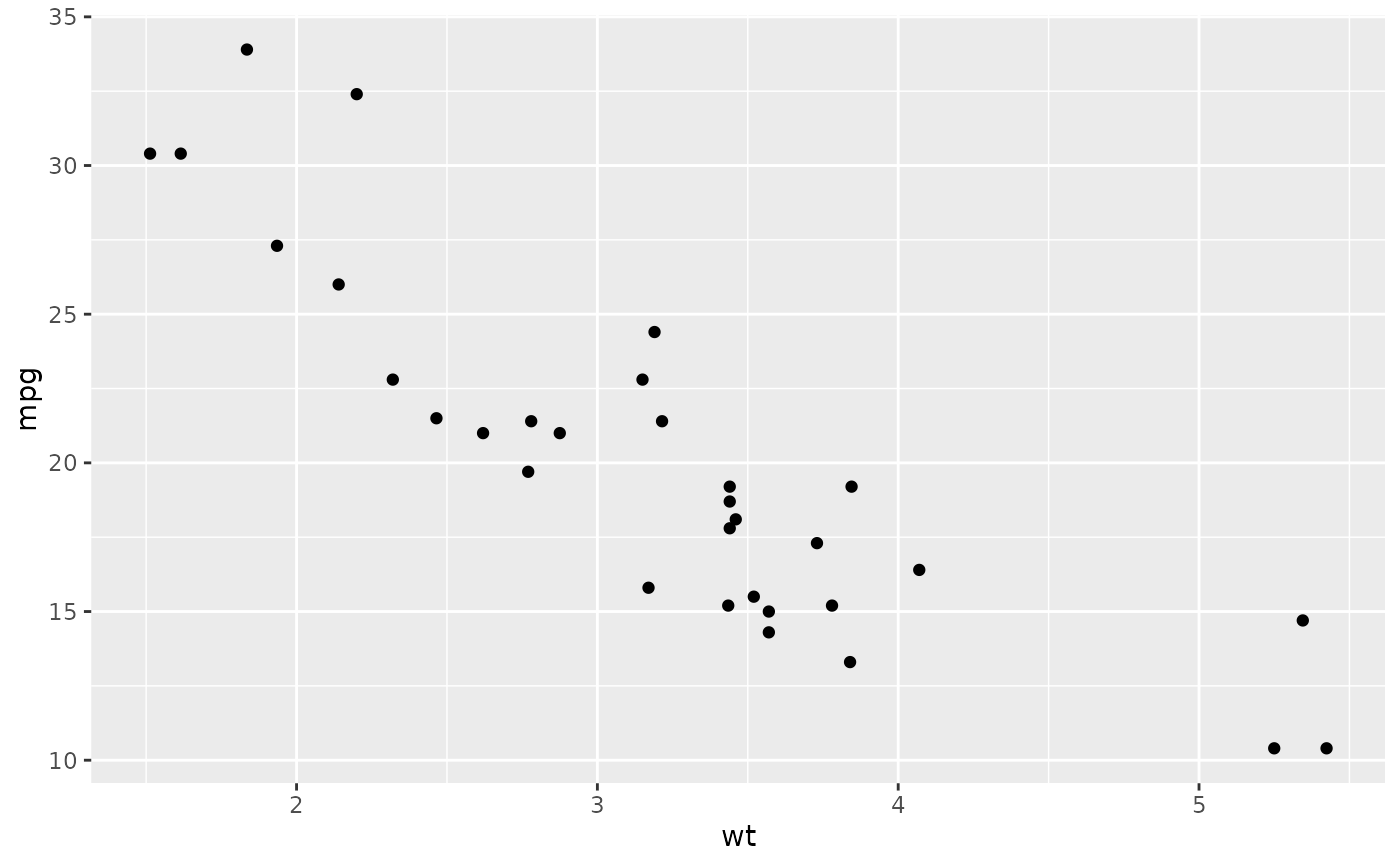p + geom_point(aes(colour = factor(cyl)))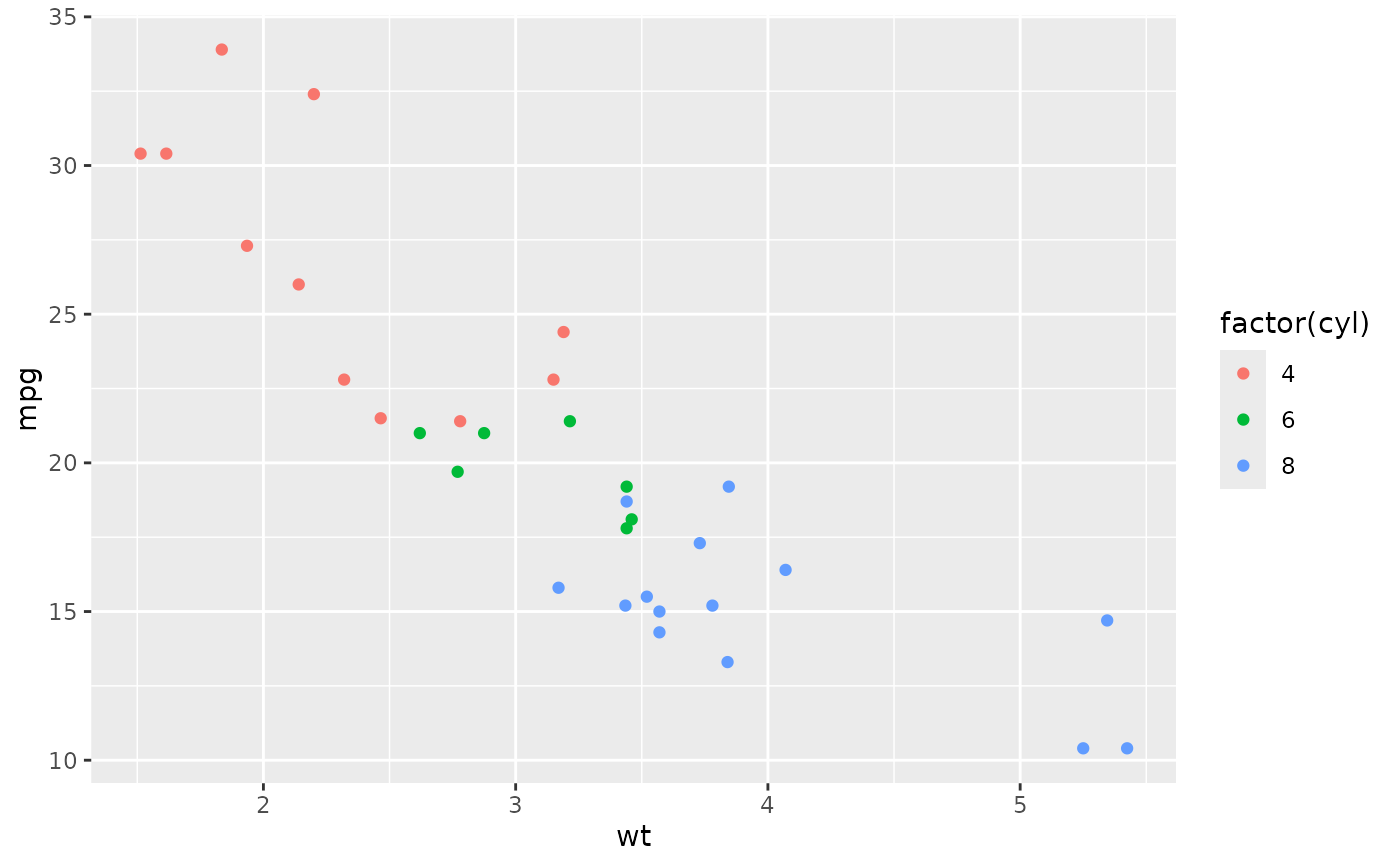p + geom_point(aes(shape = factor(cyl)))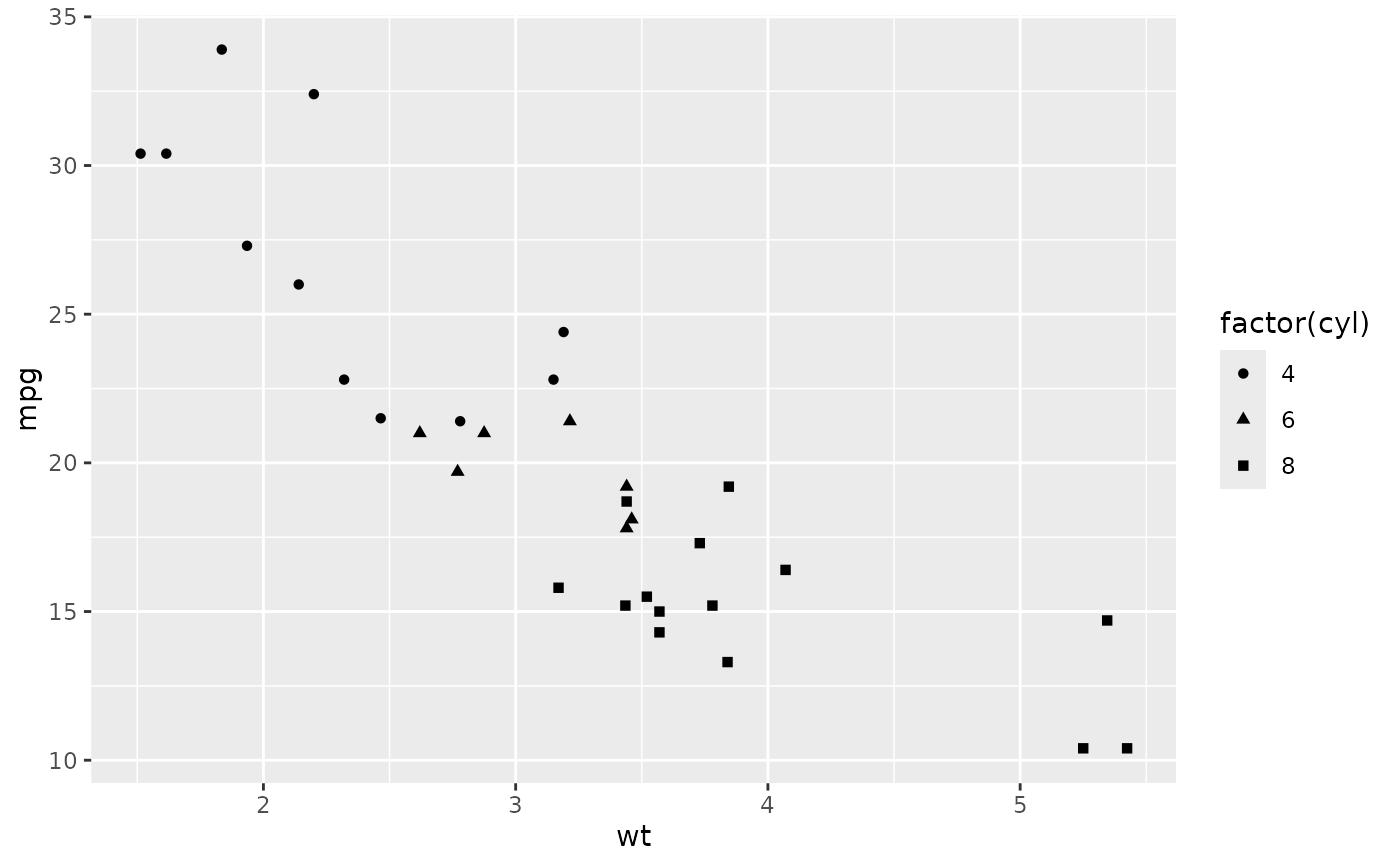# A "bubblechart":
p + geom_point(aes(size = qsec))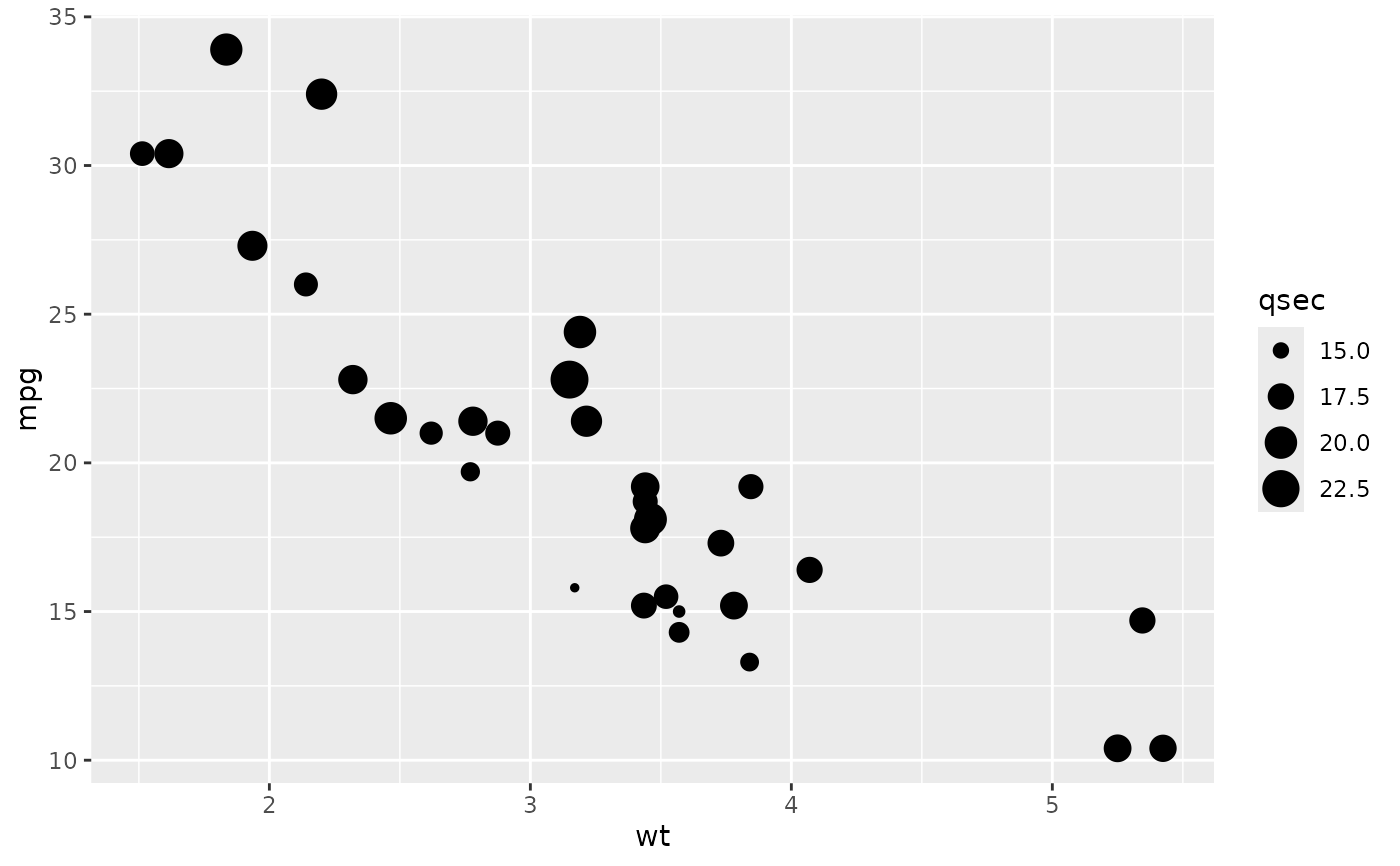# Set aesthetics to fixed value
ggplot(mtcars, aes(wt, mpg)) + geom_point(colour = "red", size = 3)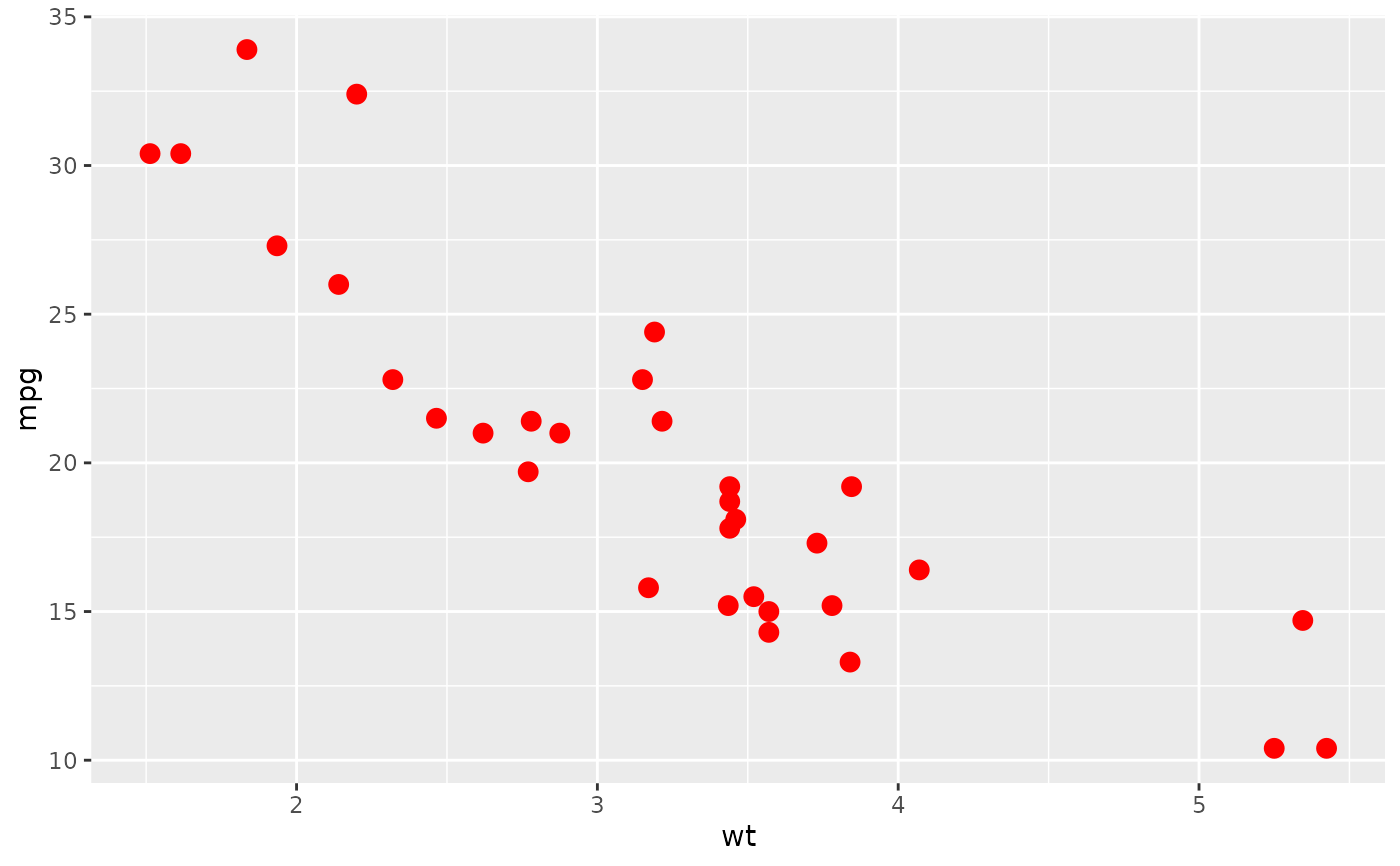# \donttest{
# Varying alpha is useful for large datasets
d <- ggplot(diamonds, aes(carat, price))
d + geom_point(alpha = 1/10)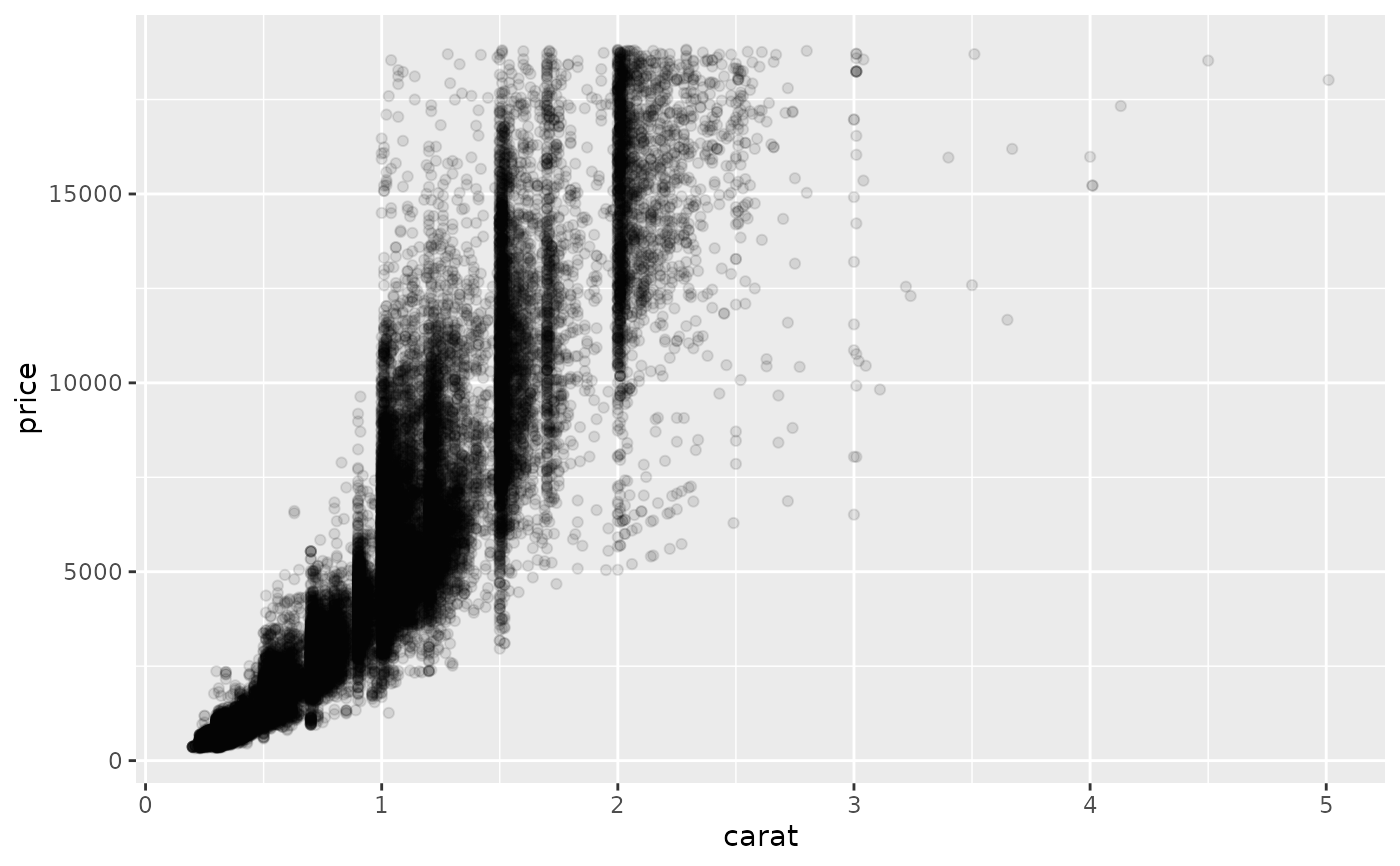d + geom_point(alpha = 1/20)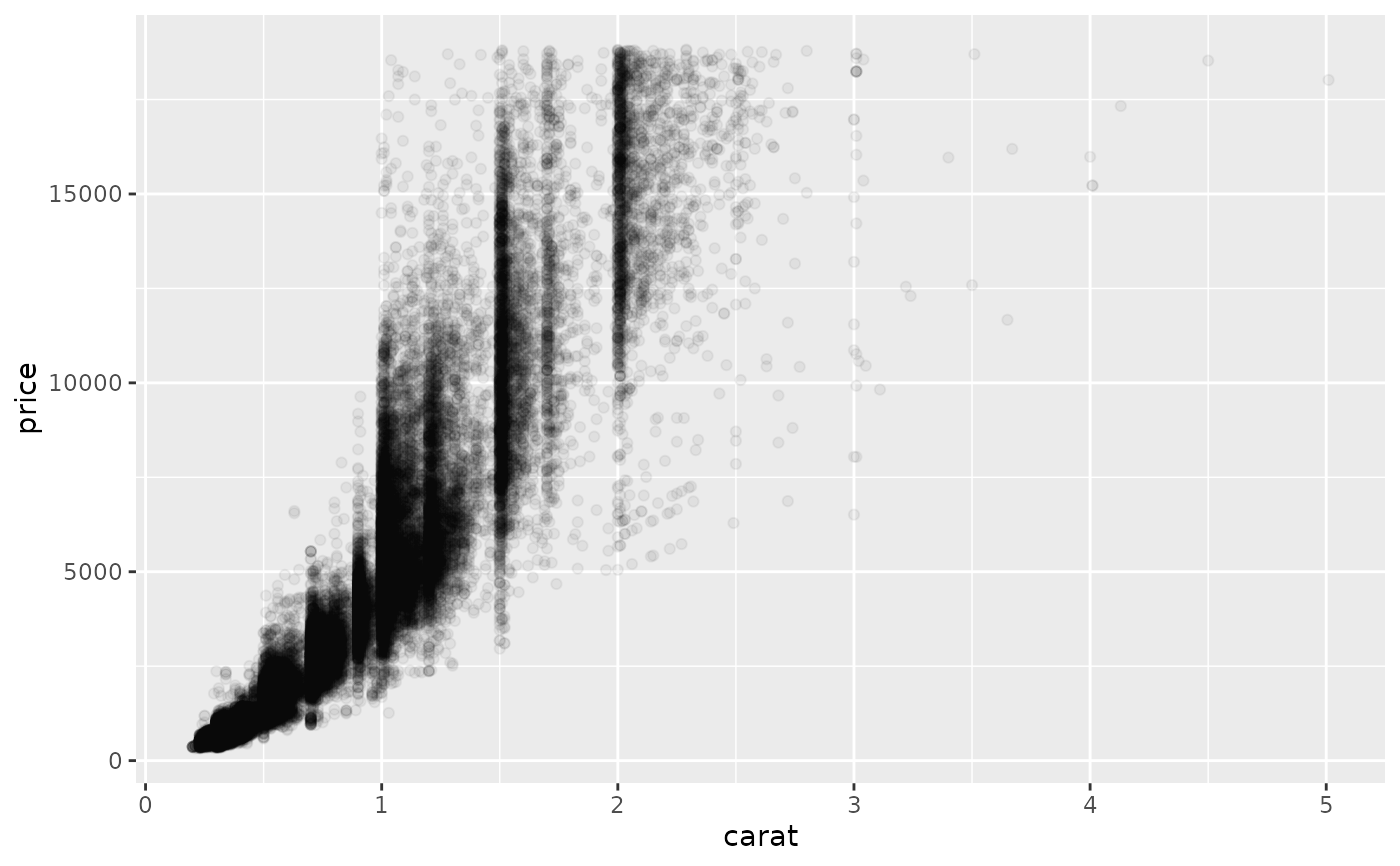d + geom_point(alpha = 1/100)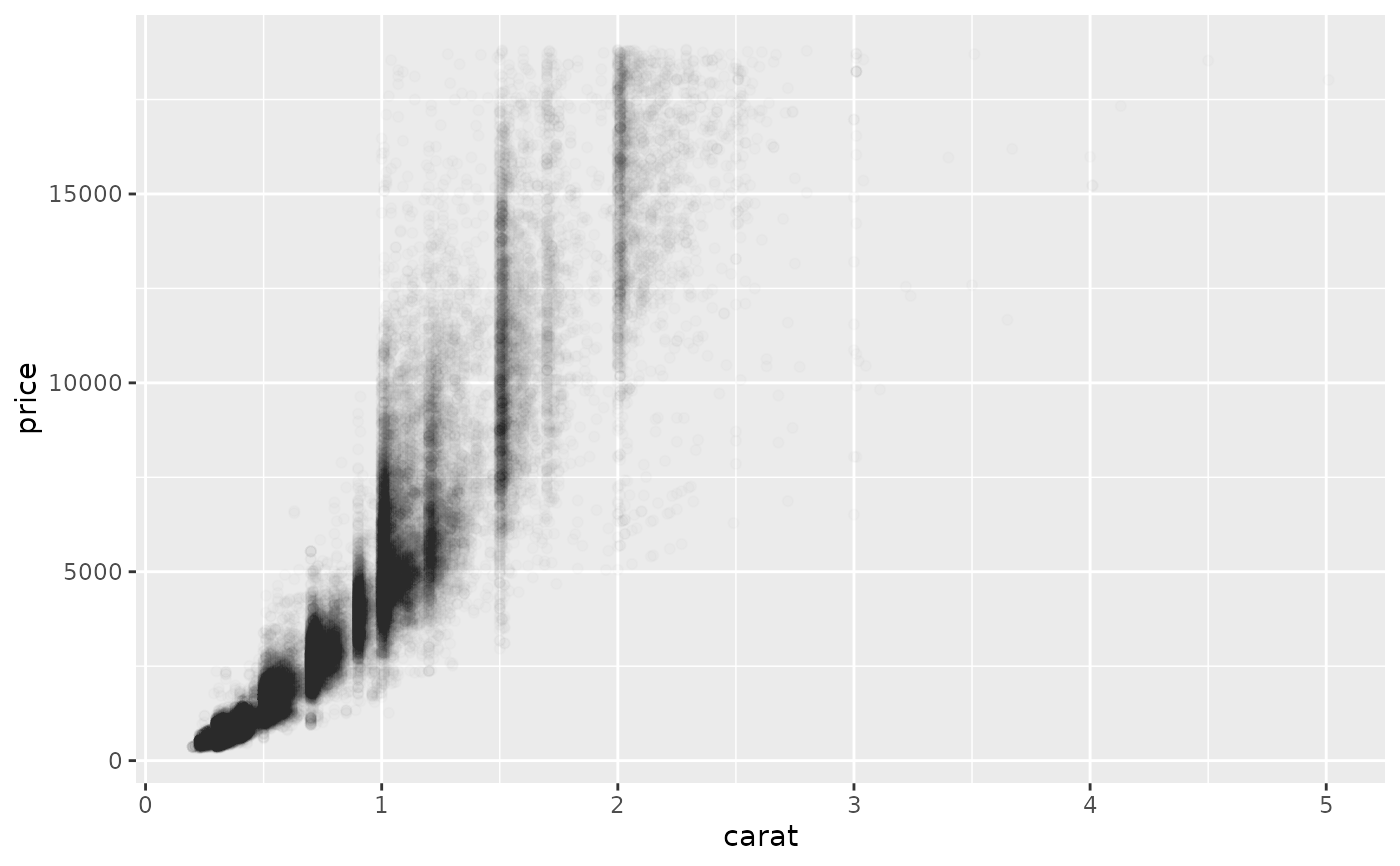# }

# For shapes that have a border (like 21), you can colour the inside and
# outside separately. Use the stroke aesthetic to modify the width of the
# border
ggplot(mtcars, aes(wt, mpg)) +
geom_point(shape = 21, colour = "black", fill = "white", size = 5, stroke = 5)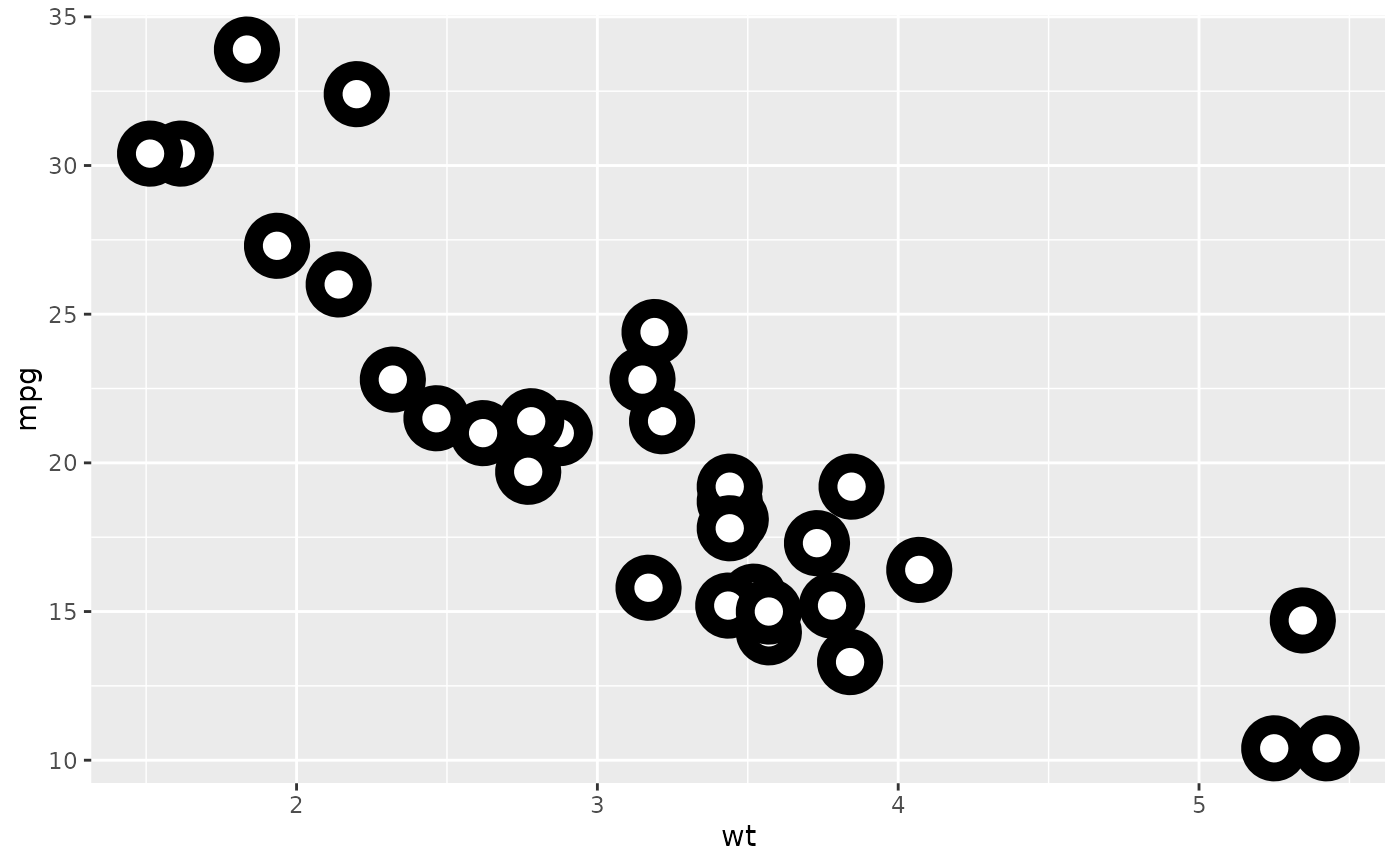# \donttest{
# You can create interesting shapes by layering multiple points of
# different sizes
p <- ggplot(mtcars, aes(mpg, wt, shape = factor(cyl)))
p +
geom_point(aes(colour = factor(cyl)), size = 4) +
geom_point(colour = "grey90", size = 1.5)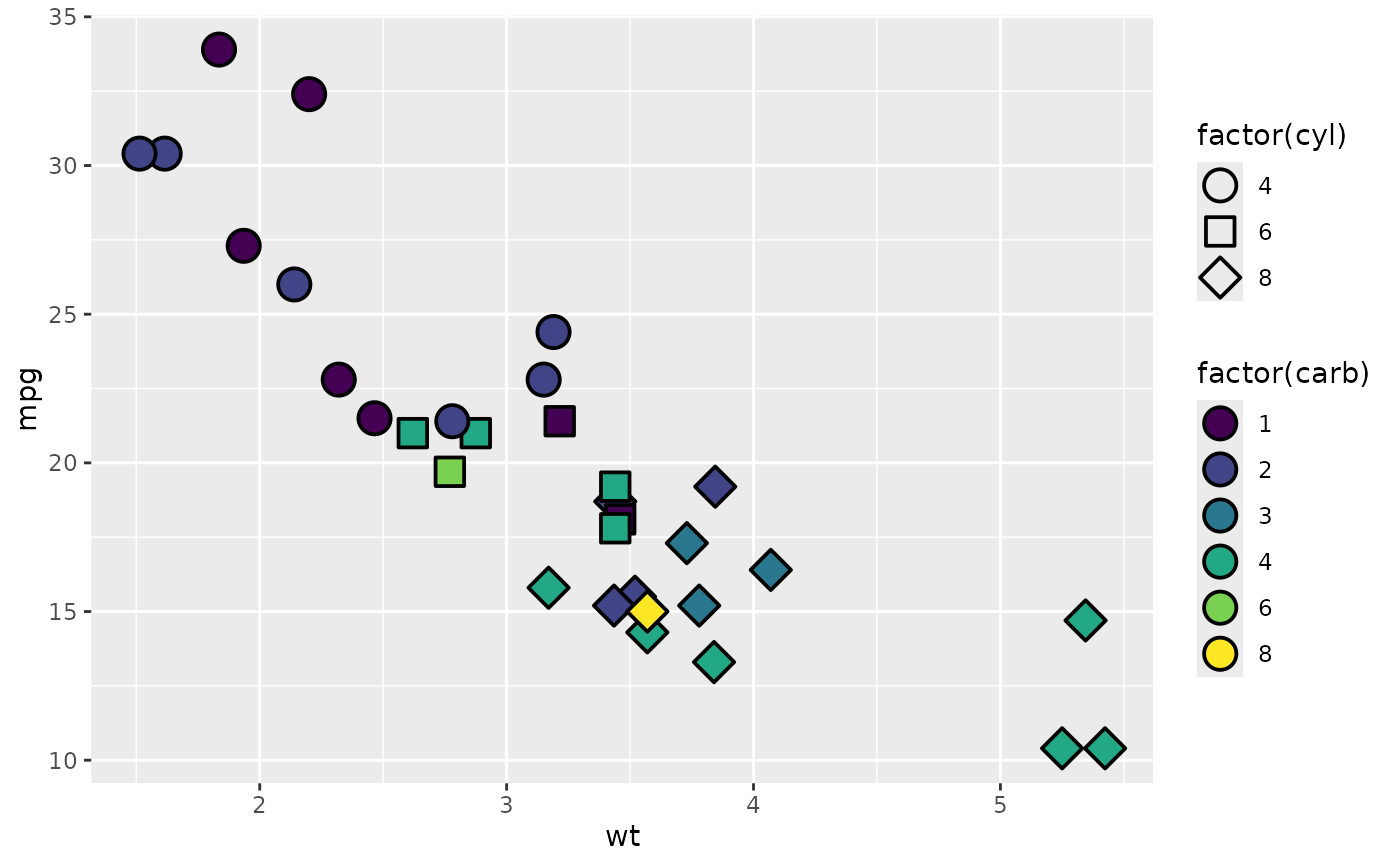p +
geom_point(colour = "black", size = 4.5) +
geom_point(colour = "pink", size = 4) +
geom_point(aes(shape = factor(cyl)))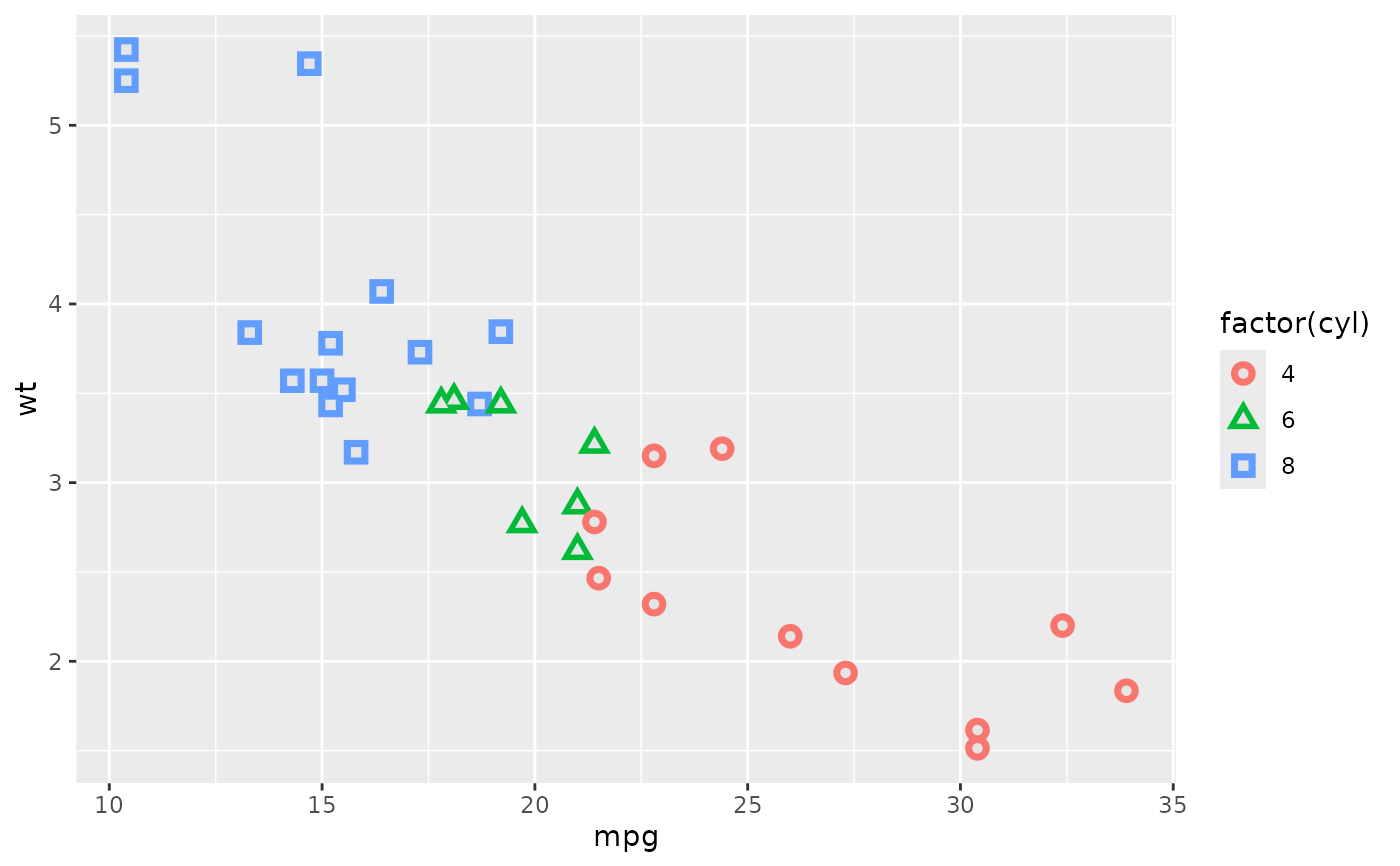# geom_point warns when missing values have been dropped from the data set
# and not plotted, you can turn this off by setting na.rm = TRUE
set.seed(1)
mtcars2 <- transform(mtcars, mpg = ifelse(runif(32) < 0.2, NA, mpg))
ggplot(mtcars2, aes(wt, mpg)) +
geom_point()
#> Warning: Removed 4 rows containing missing values or values outside the scale
#> range (geom_point()).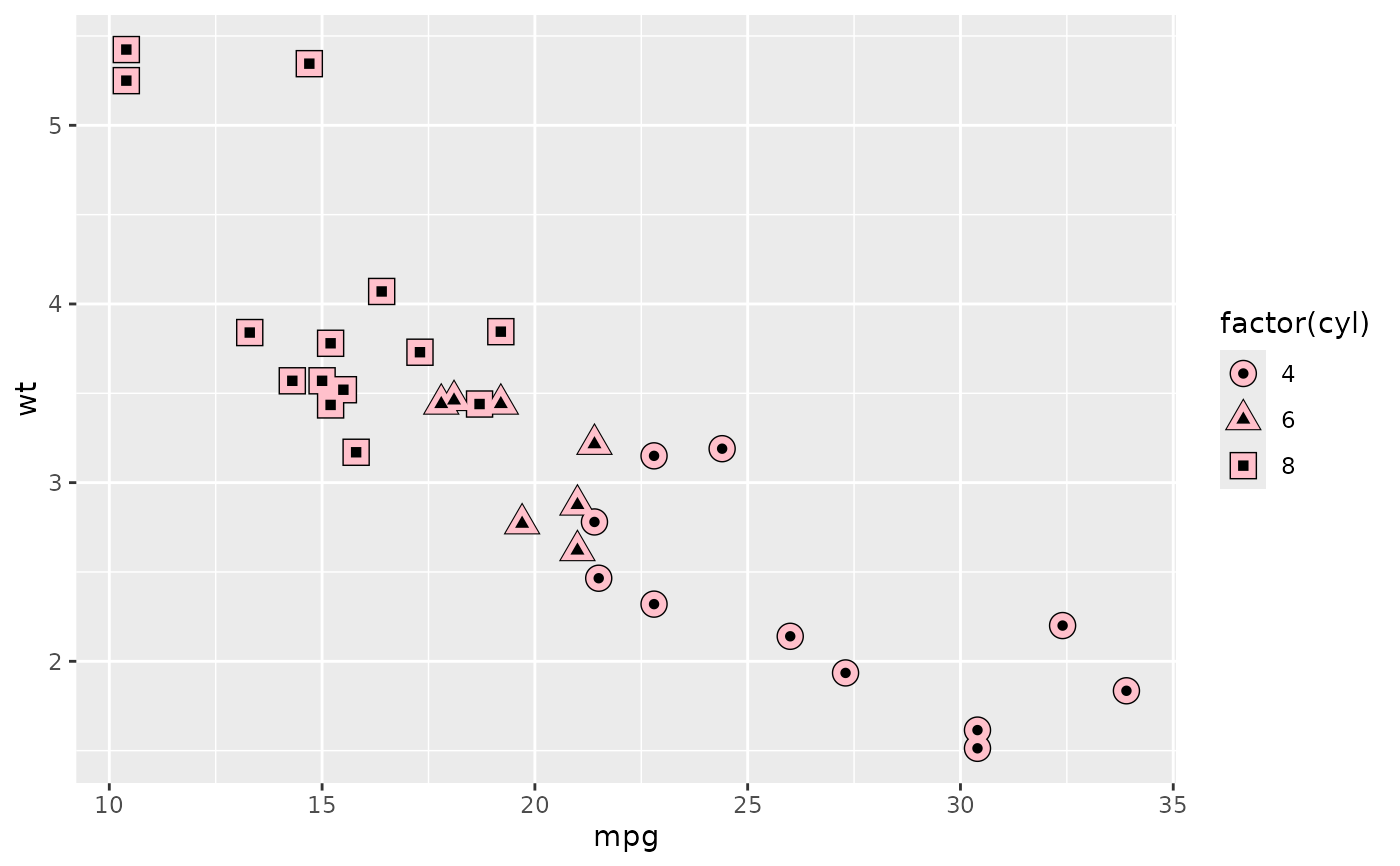ggplot(mtcars2, aes(wt, mpg)) +
geom_point(na.rm = TRUE)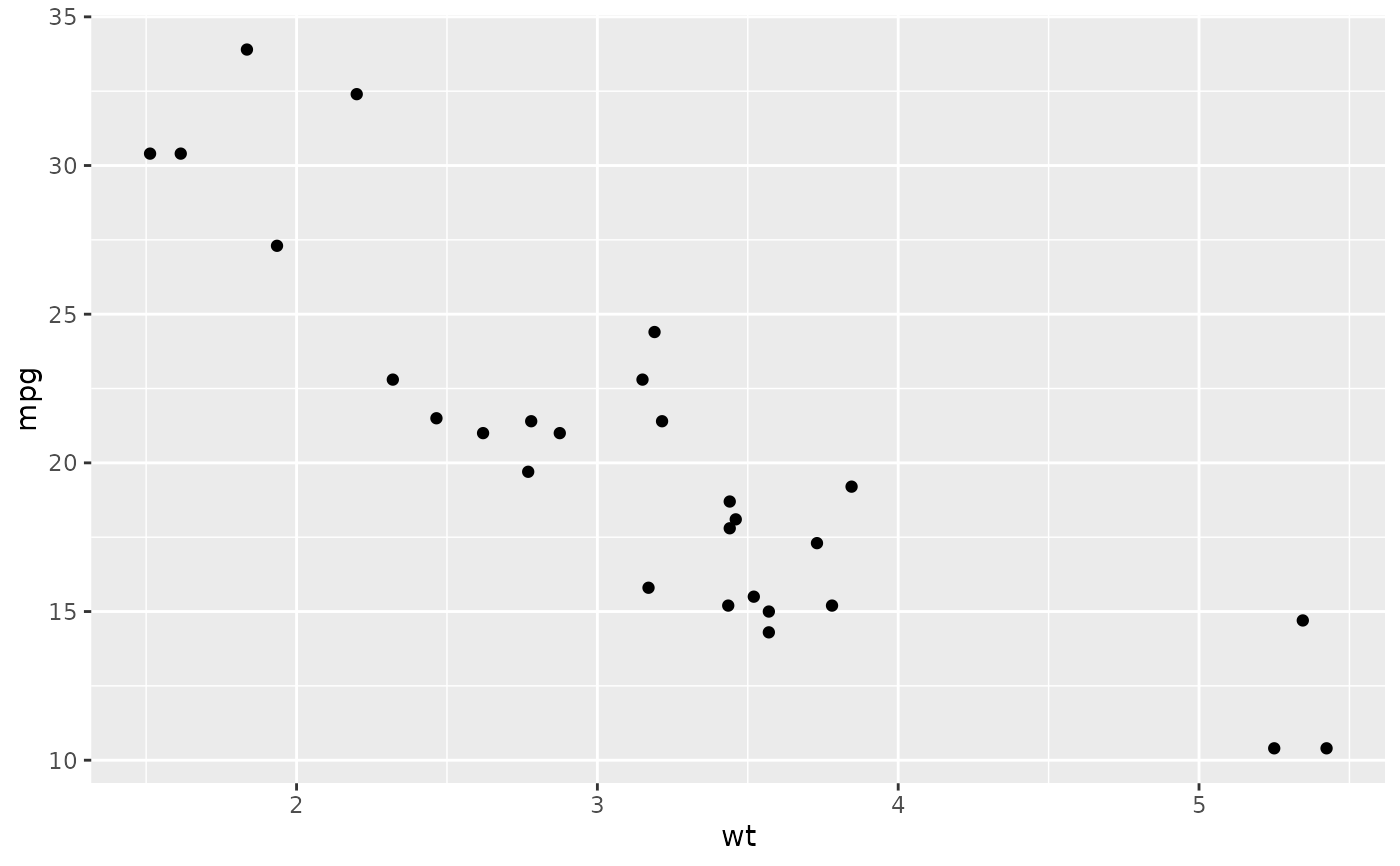# }